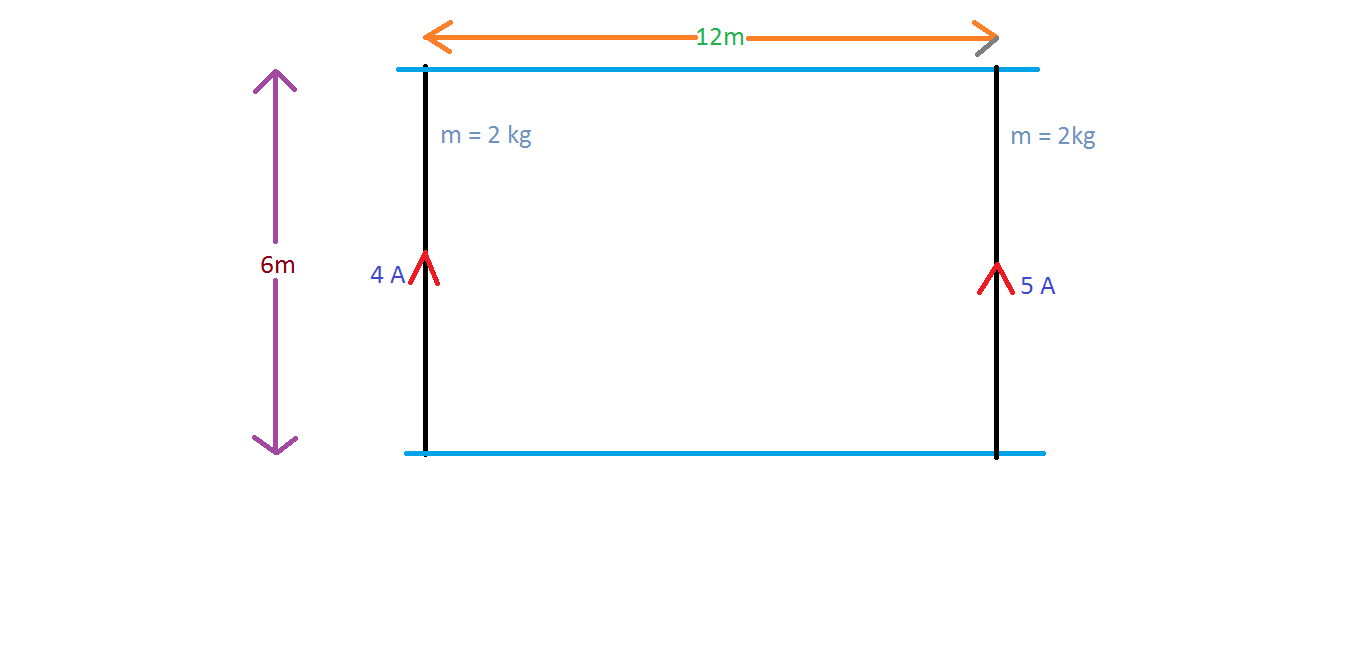# Magnetism and Mechanics

Consider two parallel wires each of mass $2 \text{ kg}$ connected to two frictionless rails separated by a distance of $6 \text{ m},$ as shown. One of them carries a current of $4 \text{ A}$ and the other carries a current of $5 \text{ A},$ both in the same direction. The wires are free to slide over the rails.

Now, the wires start moving from rest under the effect of their mutual magnetic fields. If the initial distance between the wires is $12\text{ m},$ find the velocity of the wire carrying $4 \text{ A}$ when the distance is reduced to half of its initial value.

The answer is of the form $\alpha \times {10}^{-4} \text{ m/s}.$ Find the value of $\alpha$ correct to two decimal places.×